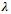Loading

# Calculate the longest and shortest wavelengths in the Balmer series of Hydrogen atom. Given Rydberg constant = 1.0987 × 107 m-1<

Publish On: 2019-05-25

# Peter

Total Post: 504

## Question: Calculate the longest and shortest wavelengths in the Balmer series of Hydrogen atom. Given Rydberg constant = 1.0987 × 107 m-1

Reply On: 2013-10-10

# lala singh

Total Post: 22

## ANS: Calculate the longest and shortest wavelengths in the Balmer series of Hydrogen atom. Given Rydberg constant = 1.0987 × 107 m-1

The wavelength () of different spectral lines of Balmer series is given by

1/= R [1/22 – 1/n22]

Longest wavelength is of Ha line or 1st line of the series. For which n2 = 3

Therefore 1/= 1.097 × 107 [1/22 – 1/32]

= 1.097 × 107 × 5/36= [36/ (5 × 1.097 × 107)] Å

For shortest wavelength, n2 = ∞

Therefore, 1/= 1.097 × 107 [1/22 – 1/∞2]

= (1.097 × 107)/ 4= (4/1.097 × 107) m

= 4 × 1010 Å /1.097 × 107= 3646 Å

#### Submit Your Answer

warning: Please Login To Submit Your Answer
Like Us On Facebook for All Latest Updates